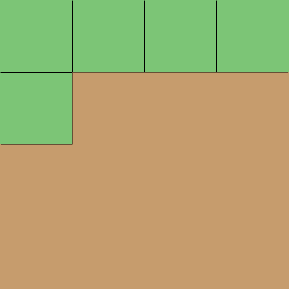SEARCH HOMEMath Central Quandaries & QueriesQuestion from Dominique, a student: The room is 12ft by 12ft. How many yards must be purchased for the carpet?Hi Dominique,

There are 3 feet in a yard so the room is 4 yards by 4 yards. Suppose the carpet came is squares, one yard by one yard so each square piece has an area of one square yard. If you cover the floor with these squares how many one square yard squares will it take?PennyMath Central is supported by the University of Regina and The Pacific Institute for the Mathematical Sciences.